login  home  contents  what's new  discussion  bug reports help  links  subscribe  changes  refresh  edit

There is are some domains in FriCAS for doing computations with non-commuting variables developed by Michel Petitot. You can find some examples in the FriCAS book under the title XPolynomial? but unfortunately the explanations are a little terse. You can also check for documentation in the source files:

and HyperDoc example page about XPOLY

In FriCAS algebra is simplified and there is no longer OrderedFreeMonoid?, we just use FreeMonoid below.

We need left and right quotients which are provided by divide to implement the substitution rules in the example below.

Test left and right exact quotients.

fricas
m1:=(x*y*y*z)$FMONOID(Symbol)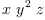(1) Type: FreeMonoid(Symbol) fricas m2:=(x*y)$FMONOID(Symbol)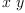(2)
Type: FreeMonoid(Symbol)
fricas
lquo(m1,m2)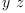(3)
Type: Union(FreeMonoid(Symbol),...)
fricas
m3:=(y*y)$FMONOID(Symbol)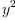(4) Type: FreeMonoid(Symbol) fricas divide(m1,m2)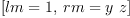(5) Type: Union(Record(lm: FreeMonoid(Symbol),rm: FreeMonoid(Symbol)),...) fricas divide(m1,m3)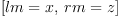(6) Type: Union(Record(lm: FreeMonoid(Symbol),rm: FreeMonoid(Symbol)),...) fricas m4:=(y^3)$FMONOID(Symbol)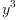(7)
Type: FreeMonoid(Symbol)
fricas
divide(m1,m4)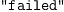(8)
Type: Union("failed",...)

This option is required to compile the functions that follow.

fricas
)set function compile on

On Tuesday, February 28, 2006 6:54 AM Fabio S. wrote:

 I would like to build the non-commutative algebra h=k[x,y] and
then I would like to make computations in h using some predefined
rules for x and y. As an example, take the three equations

x*y*x=y*x*y
x*x=a*x+b
y*y=a*y+b

where a and b are (generic, if possible) elements of k.

Then, I would like to be able to reduce polynomials in x and
y according to the previous rules. For example,

(x+y)^2 (=x^2+x*y+y*x+y^2)

should reduce to

a*(x+y)+2*b+x*y+y*x


fricas
--Generic elements of k
--OVAR = OrderedVariableList
C==>OVAR [a,b]
Type: Void
fricas
--Commutative Field: k=Q[a,b]
--Q = FRAC INT = Fration Integer
--SMP = SparseMultivariatePolynomials
K==>SMP(FRAC INT,C)
Type: Void
fricas
--Non-commutative variables
V==>OVAR [x,y]
Type: Void
fricas
--Non-commutative Algebra: h=k[x,y]
--XDPOLY XDistributedPolynomial
H==>XDPOLY(V,K)
Type: Void
fricas
--Free monoid
M==>FMONOID V
Type: Void
fricas
--Record giving result of division
Rec==>Record(lm : M, rm : M)
Type: Void
fricas
--Substitution rules are applied to words from the monoid over
--the variables and return polynomials
subs(w:M):H ==
--x*y*x=y*x*y
n:=divide(w,(x::V*y::V*x::V)$M)$M
n case Rec => monomial(1, (n::Rec).lm)$H * (y::V*x::V*y::V)$H * monomial(1, (n::Rec).rm)$H --x*x=a*x+b n:=divide(w,(x::V^2)$M)$M n case Rec => monomial(1, (n::Rec).lm)$H * (a::K*x::V+b::K)$H * monomial(1, (n::Rec).rm)$H
--y*y=a*y+b
n:=divide(w,(y::V^2)$M)$M
n case Rec => monomial(1, (n::Rec).lm)$H * (a::K*y::V+b::K)$H * monomial(1, (n::Rec).rm)$H --no change monomial(1, w)$H
Function declaration subs : FreeMonoid(OrderedVariableList([x,y]))
-> XDistributedPolynomial(OrderedVariableList([x,y]),
SparseMultivariatePolynomial(Fraction(Integer),
OrderedVariableList([a,b]))) has been added to workspace.
Type: Void
fricas
--Apply rules to a term. Keep coefficients
newterm(x:Record(k:M,c:K)):H==x.c*subs(x.k)
Function declaration newterm : Record(k: FreeMonoid(
OrderedVariableList([x,y])),c: SparseMultivariatePolynomial(
Fraction(Integer),OrderedVariableList([a,b]))) ->
XDistributedPolynomial(OrderedVariableList([x,y]),
SparseMultivariatePolynomial(Fraction(Integer),
OrderedVariableList([a,b]))) has been added to workspace.
Type: Void
fricas
--Reconstruct polynomial, term-by-term
newpoly(t:H):H==reduce(+,map(newterm,listOfTerms(t)))
Function declaration newpoly : XDistributedPolynomial(
OrderedVariableList([x,y]),SparseMultivariatePolynomial(Fraction(
Integer),OrderedVariableList([a,b]))) -> XDistributedPolynomial(
OrderedVariableList([x,y]),SparseMultivariatePolynomial(Fraction(
workspace.
Type: Void

Example calculations:

fricas
p1:=(x::V+y::V)$H^2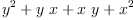(9) Type: XDistributedPolynomial(OrderedVariableList([x,y]),SparseMultivariatePolynomial?(Fraction(Integer),OrderedVariableList([a,b]))) fricas newpoly(p1) fricas Compiling function newpoly with type XDistributedPolynomial( OrderedVariableList([x,y]),SparseMultivariatePolynomial(Fraction( Integer),OrderedVariableList([a,b]))) -> XDistributedPolynomial( OrderedVariableList([x,y]),SparseMultivariatePolynomial(Fraction( Integer),OrderedVariableList([a,b]))) fricas Compiling function subs with type FreeMonoid(OrderedVariableList([x, y])) -> XDistributedPolynomial(OrderedVariableList([x,y]), SparseMultivariatePolynomial(Fraction(Integer), OrderedVariableList([a,b]))) fricas Compiling function newterm with type Record(k: FreeMonoid( OrderedVariableList([x,y])),c: SparseMultivariatePolynomial( Fraction(Integer),OrderedVariableList([a,b]))) -> XDistributedPolynomial(OrderedVariableList([x,y]), SparseMultivariatePolynomial(Fraction(Integer), OrderedVariableList([a,b])))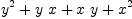(10) Type: XDistributedPolynomial(OrderedVariableList([x,y]),SparseMultivariatePolynomial?(Fraction(Integer),OrderedVariableList([a,b]))) fricas p2:=(x::V+y::V)$H^3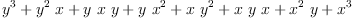(11)
Type: XDistributedPolynomial(OrderedVariableList([x,y]),SparseMultivariatePolynomial?(Fraction(Integer),OrderedVariableList([a,b])))
fricas
newpoly(p2)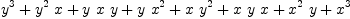(12)
Type: XDistributedPolynomial(OrderedVariableList([x,y]),SparseMultivariatePolynomial?(Fraction(Integer),OrderedVariableList([a,b])))

newpoly above applies rules once. However the rules above should be applied more than once - they should be applied until no more changes are possible. This is done below:

fricas
reduce(p:H):H ==
p2 := newpoly(p)
p3 := newpoly(p2)
while p3 ~= p2 repeat
p2 := p3
p3 := newpoly(p2)
p3
Function declaration reduce : XDistributedPolynomial(
OrderedVariableList([x,y]),SparseMultivariatePolynomial(Fraction(
Integer),OrderedVariableList([a,b]))) -> XDistributedPolynomial(
OrderedVariableList([x,y]),SparseMultivariatePolynomial(Fraction(
workspace.
Compiled code for newpoly has been cleared.
Type: Void
fricas
reduce(p2)
fricas
Compiling function newpoly with type XDistributedPolynomial(
OrderedVariableList([x,y]),SparseMultivariatePolynomial(Fraction(
Integer),OrderedVariableList([a,b]))) -> XDistributedPolynomial(
OrderedVariableList([x,y]),SparseMultivariatePolynomial(Fraction(
Integer),OrderedVariableList([a,b])))
fricas
Compiling function reduce with type XDistributedPolynomial(
OrderedVariableList([x,y]),SparseMultivariatePolynomial(Fraction(
Integer),OrderedVariableList([a,b]))) -> XDistributedPolynomial(
OrderedVariableList([x,y]),SparseMultivariatePolynomial(Fraction(
Integer),OrderedVariableList([a,b])))(13)
Type: XDistributedPolynomial(OrderedVariableList([x,y]),SparseMultivariatePolynomial?(Fraction(Integer),OrderedVariableList([a,b])))

 Subject:   Be Bold !! ( 14 subscribers )